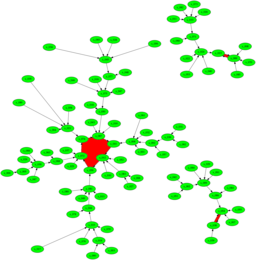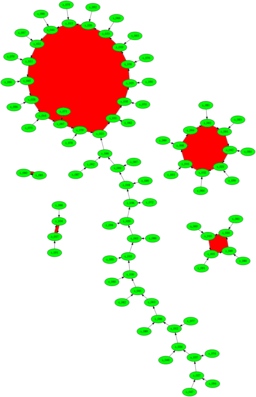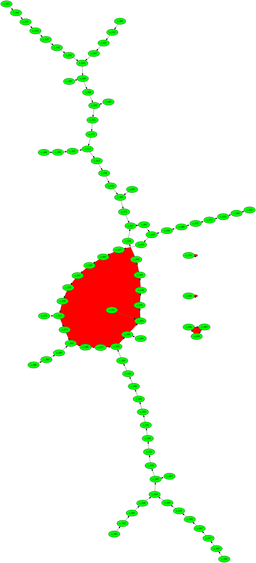# Idle Thoughts About Pollard RhoSubscribe!My latest posts can be found here:
Previous blog posts:
 This post will be less polished, as I'm not really sure what I want to say, but thought I'd get it "out there" to see if anyone had any comments.

## Pollard Rho in brief

The basic idea is that we have a number $N$ to factor, and we've already stripped out all the really small factors by using a quick hit of trial division. Now what we do is run around the integers modulo N and hope to get a coincidence.

So we have $N$ and we assume that there is some $p,$ currently unknown to us, which divides $N.$

We're trying to find $p.$

We define a sequence $\{x_i\}_{i=0}^\infty$ with $x_0$ something a bit arbitrary, and $x_{i+1}=x_i^2+c$ for some $c,$ often just a small integer from 1 to 5 or so.

We compute the sequence modulo $N,$ but thinking about it modulo $p$ we can see that it must eventually start repeating. So after some values that don't get repeated we end up in a cycle (mod $p$).

So at some point $x_i=x_j\ (mod p)$ and we can detect this by computing $gcd(x_j-x_i,N)$ and finding that it's bigger than 1.

## The Algorithm

So the algorithm is:


def f(x): x^2 + c

x_0 = k:
rabbit = x_0
turtle = x_0
while True:
rabbit = f(f(rabbit))
turtle = f(turtle)
g = gcd(rabbit-turtle,N)
if 1 < g <=N:
return gN=93, c=5

## Why it works

So how and why does this work?

Thinking about the sequence ${x}$ mod $p$ we can see that it eventually cycles. Each term is the square of the one before, plus a constant, so we can think of the values as "pinging about randomly," and because of the birthday paradox says we should get a repeat time $O(\sqrt{p}).$

So if $p$ isn't too big we should find it reasonably quickly, and in any rate in time $O(n^{\frac{1}{4}}).$

## And now for something completely different

So why do we use $f(x)=x^2+c$ ? Is there something special about that?

If we have any pseudo-random $f:Z_p -> Z_p$ then we'd think that would work. So we plot the digraph with nodes for integers and edges for $a$ goes to $b,$ the digraph we get for the quadratic shows that there are lots of cycles that aren't too large, and the paths that take you into the cycles are not very long.

On the other hand, the first quick hack I tried for a pseudo-random thingy either has very long tails before we get to a cycle, or has very long cycles.I don't understand this.

True, I've only drawn digraphs for comparatively small $N,$ because I don't have the tools immediately to hand to layout huge graphs. But even so, there's something going on here that I don't understand.

I suspect there's something going on with the fact that $x^2+c$ is such that every node has out degree 1, but in degree either 0 or 2 (with a couple of possible exceptions). That might have something to do with the overall structure, but I don't see how that's critical.

And if it is critical, then perhaps we can make a pseudo-random function that has the right properties, but is faster than computing the square each time. It's not a huge speed-up, but it might be worth having.

And if it is the degree structure that matters, perhaps we can devise one that's fast to compute and even more favourable.

As I say, idle musings ...

## References

 <<<< Prev <<<< When Optimising Code Measure : >>>> Next >>>> More Musing On Pollard Rho ...You can follow me on Mathstodon.

 Of course, you can alsofollow me on twitter:## Send us a comment ...

 You can send us a message here. It doesn't get published, it just sends us an email, and is an easy way to ask any questions, or make any comments, without having to send a separate email. So just fill in the boxes and then: If you include an email address then I'll be able to respond, and conversely, if you don't, I won't! Likewise, if you give me explicit permission to add your comments here then I might choose to do so, and if you don't, I won't! So if you reply, please include your preferences, otherwise I'll read you email, nod sagely, and file it away.

 Your name : Email : Message :

# ContentsSuggest a change ( <-- What does this mean?) / Send me email